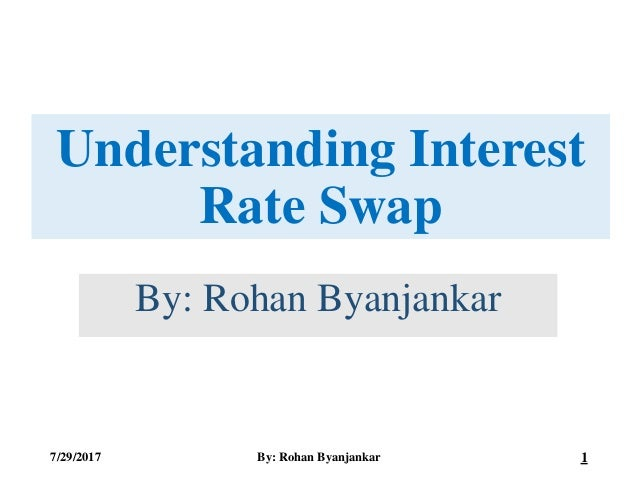Successfully reported this slideshow.Upcoming SlideShare
×

# Understanding Interest Rate Swap: Price of Interest Rate Swap and Value of Interest Rate Swap

749 views

Published on

Meaning of Interest Rate Swap, Types of Interest Rate Swap, Pricing of Interest Rate Swap and Value of Interest Rate Swap

Published in: Education
• Full Name
Comment goes here.

Are you sure you want to Yes No• Be the first to comment

### Understanding Interest Rate Swap: Price of Interest Rate Swap and Value of Interest Rate Swap

1. 1. Understanding Interest Rate Swap By: Rohan Byanjankar 7/29/2017 By: Rohan Byanjankar 1
2. 2. Interest Rate Swap • Interest Rate Swap is one the types of Swap Contract in which two parties with equal principal amount called notional principal agrees to exchange their cash flow for a specified period of time. • Two parties can either enter into interest rate swap contract: • To exchange fixed and floating interest cash flows, which is called Plain Vanilla Swap • To exchange both floating interest cash flows, which is called Basis Swap 7/29/2017 By: Rohan Byanjankar 2
3. 3. Price of Swap • The price of a swap is the coupon rate that makes the fixed rate bond to have a value equal to that of the floating rate bond, and thus causes the initial swap value to equal zero. 7/29/2017 By: Rohan Byanjankar 3
4. 4. Pricing of Swap • Price of Swap = 1 q × 1−𝑃𝑉𝐼𝐹(𝑛) 1 n PVIF(i) • Where, • q = Adjustment Factor • PVIF(n) = Present Value factor of nth term • 1 n PVIF(i) = Sum of Present Value factor of all ith term, where i tends to 1 to n. 7/29/2017 By: Rohan Byanjankar 4
5. 5. Value of Swap • Value of Swap is the net gain or loss to the parties involved in swap contract. • It is the difference between present value of two streams of cash flow. • Value of Swap is obtained by calculating the difference between Value of Floating Rate Bond and Value of Fixed Rate Bond. 7/29/2017 By: Rohan Byanjankar 5
6. 6. Value of Plain Vanilla Swap Plain Vanilla Swap • If a party is paying fixed rate and receiving floating rate, then • Value of Swap (Vs) = Value of Floating Rate Bond (V(FLRB)) - Value of Fixed Rate Bond (V(FXRB)) • If Vs>0, Party receiving fixed rate is in loss • If Vs<0, Party receiving floating rate is in loss. 7/29/2017 By: Rohan Byanjankar 6
7. 7. Value of Floating Rate Swap V FLRB = Principal + Interest × PVIF(1) V(FLRB) = NP + NP × R FL × q × PVIF(1) Here, NP = Notional Principal R(FL) = Floating Interest Rate NP × R FL × q = Interest (I) PVIF(1) = Present Value factor of 1st term 7/29/2017 By: Rohan Byanjankar 7 Value of Plain Vanilla Swap
8. 8. Value of Fixed Rate Swap V(FXRB) = Sum of Present Value of Interest + Present Value of Face Value or NP V FXRB = NP × R FX × q × 1 n PVIF(i) + NP × PVIF n Here, R(FX) = Fixed Rate of Bond 7/29/2017 By: Rohan Byanjankar 8 Value of Plain Vanilla Swap
9. 9. Spread = Price of LIBOR Swap – Price of T_Bill Rate Swap Note: Spread is either deducted from LIBOR or is added to T-Bill Rate while calculating Value of Basis Swap 7/29/2017 By: Rohan Byanjankar 9 Spread in Basis Swap
10. 10. 7/29/2017 By: Rohan Byanjankar 10 Value of Basis Swap Basis Swap • If a party is paying T-bill rate and receiving LIBOR, then • Value of Swap (Vs) = Value of LIBOR Floating Rate Bond (VL(FLRB)) - Value of T-Bill Floating Rate Bond (VT(FXRB)) • If Vs>0, Party receiving T-bill rate is in loss • If Vs<0, Party receiving LIBOR is in loss.
11. 11. 7/29/2017 By: Rohan Byanjankar 11 Value of LIBOR Rate Swap VL FLRB = Principal + Interest × PVIF(1) VL(FLRB) = NP + NP × (R LFL − Spread) × q × PVIF(1) Here, NP = Notional Principal R(LFL) = Original LIBOR Floating Interest Rate of 1st Term NP × R FL × q = Interest (I) PVIF(1) = Present Value factor of 1st term Value of Basis Swap
12. 12. 7/29/2017 By: Rohan Byanjankar 12 Value of T-bill Rate Swap VT FLRB = Principal + Interest × PVIF(1) VT(FLRB) = NP + NP × R TFL × q × PVIF(1) Here, NP = Notional Principal R(TFL) = Original T-Bill Floating Rate of 1st Term NP × R FL × q = Interest (I) PVIF(1) = Present Value factor of 1st term Value of Basis Swap